Select Page

# Thermodynamics Cycles

Thermodynamics Cycles

Contents

Recall the working principle of the Otto cycle with the help of p-v and T-s diagrams 1

Calculate the following parameters of the Otto cycle: i. Thermal Efficiency ii. Work done iii. Mean effective pressure iv. Compression ratio 4

Recall the working principle of the Diesel cycle with the help of p-v and T-s diagrams 5

Calculate the following parameters of the Diesel cycle: i. Thermal Efficiency ii. Work done iii. Mean effective pressure 7

Recall the working principle of Dual cycle with the help of p-v and T-s diagram 8

Recall the comparison between Otto, Diesel, and Dual Cycle 10

Recall the working principle of Reversed Carnot cycle with the help of p-v and T-s diagrams 11

Recall the working principle of Bell Coleman or Reversed Brayton cycle with the help of p-v and T-s diagrams 13

Derive an expression for COP of the Bell Coleman cycle 15

Recall the working principle of the Rankine cycle with the help of p-v and T-s diagrams 16

Derive the expression for the Efficiency of the Rankine cycle 18

# Recall the working principle of the Otto cycle with the help of p-v and T-s diagrams

The Otto cycle is a type of thermodynamic cycle that describes the operation of a four-stroke internal combustion engine, such as the one used in most vehicles. The cycle consists of four processes: intake, compression, power, and exhaust. The cycle is named after Nikolaus Otto, who invented the four-stroke engine.

The working principle of the Otto cycle can be understood with the help of pressure-volume (p-v) diagrams and temperature-entropy (T-s) diagrams.

In the intake process, the piston moves down and a mixture of fuel and air is drawn into the cylinder. This process is known as the suction stroke and occurs at a constant volume. The pressure and temperature of the gas in the cylinder decrease during this process.

In the compression process, the piston moves up and compresses the fuel-air mixture. This process occurs at a constant temperature and the pressure and temperature of the gas in the cylinder increase.

In the power stroke, the compressed fuel-air mixture is ignited by a spark and undergoes a rapid expansion. This process is exothermic and releases a large amount of heat. The pressure and temperature of the gas in the cylinder increase further, causing the piston to move down and perform work.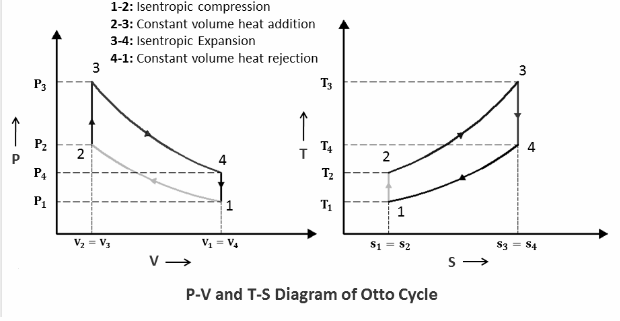In the exhaust process, the piston moves up and the exhaust gases are expelled from the cylinder. This process occurs at a constant volume and the pressure and temperature of the gas in the cylinder decrease.

The Otto cycle is represented on a pressure-volume diagram as a cycle that traces out a closed loop, with the four processes represented by four distinct points on the loop. On a temperature-entropy diagram, the Otto cycle is represented by a cycle that also traces out a closed loop, with the four processes represented by four distinct points on the loop.

In conclusion, the Otto cycle is a fundamental concept in thermodynamics and is used to understand the operation of internal combustion engines. The cycle can be represented on p-v and T-s diagrams, which provide valuable insights into the thermodynamic behavior of the engine and the relationships between different thermodynamic properties.

# Calculate the following parameters of the Otto cycle: i. Thermal Efficiency ii. Work done iii. Mean effective pressure iv. Compression ratio

The Otto cycle is a thermodynamic cycle that describes the operation of a four-stroke internal combustion engine. There are several parameters that can be calculated to characterise the performance of the Otto cycle, including thermal efficiency, work done, mean effective pressure, and compression ratio.

1. Thermal Efficiency: The thermal efficiency of the Otto cycle is a measure of the amount of energy that is converted into useful work by the engine. It is calculated as the ratio of the work done by the engine to the heat added to the cycle, and is expressed as a percentage. The thermal efficiency of an Otto cycle can be calculated using the following formula:
η = (W / Qin) * 100%

Where W is the work done by the engine and Qin is the heat added to the cycle.

1. Work done: The work done by the engine during the Otto cycle is the amount of energy that is converted into useful work. It can be calculated using the following formula:
W = m * R * T * ln(V2 / V1)

Where m is the mass of the working fluid, R is the ideal gas constant, T is the temperature of the working fluid, V1 is the volume at the beginning of the cycle, and V2 is the volume at the end of the cycle.

1. Mean effective pressure: The mean effective pressure (MEP) is a measure of the average pressure exerted by the engine over one cycle. It is calculated as the average of the maximum and minimum pressures in the cylinder during the cycle. The MEP is an important parameter in the design of internal combustion engines and is used to determine the size and power of the engine.
2. Compression ratio: The compression ratio of an Otto cycle is the ratio of the volume of the cylinder at the end of the compression process to the volume of the cylinder at the beginning of the compression process. It is a measure of the degree of compression of the fuel-air mixture in the cylinder and is an important parameter in the design of internal combustion engines. The compression ratio can be calculated using the following formula:
r = Vmax / Vmin

Where Vmax is the maximum volume of the cylinder and Vmin is the minimum volume of the cylinder.

In conclusion, these parameters are key measures of the performance of an Otto cycle and provide valuable insights into the thermodynamic behaviour of internal combustion engines. The thermal efficiency, work done, mean effective pressure, and compression ratio are all important considerations in the design and optimization of internal combustion engines.

# Recall the working principle of the Diesel cycle with the help of p-v and T-s diagrams

The Diesel cycle is a thermodynamic cycle that describes the operation of a four-stroke internal combustion engine, named after the German inventor Rudolf Diesel. The working principle of the Diesel cycle is based on the compression of air in the cylinder to a high pressure, followed by the injection of fuel into the compressed air, which ignites and drives the engine. The Diesel cycle is characterized by its high compression ratio, which allows for a more efficient conversion of heat into useful work.

The Diesel cycle can be represented on a pressure-volume (p-v) diagram and a temperature-entropy (T-s) diagram. The p-v diagram shows the pressure and volume of the working fluid in the engine over one cycle, while the T-s diagram shows the temperature and entropy of the working fluid over one cycle.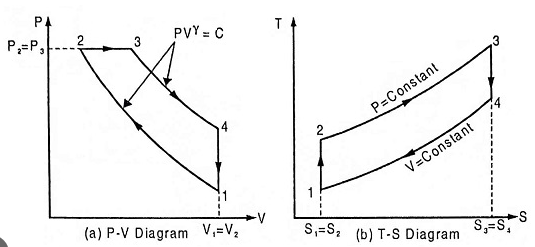In the Diesel cycle, the four strokes of the engine are:

1. Intake stroke: During this stroke, the piston moves downward and air is drawn into the cylinder. The pressure in the cylinder decreases and the volume increases.
2. Compression stroke: During this stroke, the piston moves upward and compresses the air in the cylinder. The pressure and temperature of the air increase, while the volume decreases.
3. Combustion stroke: During this stroke, fuel is injected into the cylinder and ignites, driving the engine. The pressure and temperature of the working fluid increase, while the volume remains constant.
4. Exhaust stroke: During this stroke, the piston moves downward and the exhaust gases are expelled from the cylinder. The pressure and temperature of the working fluid decrease, while the volume increases.

In conclusion, the Diesel cycle is a thermodynamic cycle that describes the operation of a four-stroke internal combustion engine. The high compression ratio and efficient conversion of heat into useful work make the Diesel cycle an important consideration in the design and optimization of internal combustion engines. The p-v and T-s diagrams are useful tools for visualizing and understanding the thermodynamic behavior of the Diesel cycle.

# Calculate the following parameters of the Diesel cycle: i. Thermal Efficiency ii. Work done iii. Mean effective pressure

The Diesel cycle is a thermodynamic cycle that describes the operation of a four-stroke internal combustion engine, and it is characterized by its high compression ratio and efficient conversion of heat into useful work. To understand the performance of the Diesel cycle, it is important to calculate certain parameters that describe its thermodynamic behavior. These parameters include:

1. Thermal Efficiency: Thermal efficiency is the ratio of the useful work done by the engine to the amount of heat added to the working fluid. It is a measure of how efficiently the engine converts heat into useful work, and it is an important factor in determining the fuel efficiency of the engine. The thermal efficiency of the Diesel cycle can be calculated using the formula:

η = (Wout / Qin) * 100%

Where Wout is the work done by the engine, and Qin is the heat added to the working fluid.

1. Work done: Work done is the amount of useful work performed by the engine over one cycle. It is an important parameter in determining the engine’s power output. The work done in the Diesel cycle can be calculated using the formula:

Wout = (V2 – V1) * Pavg

Where V1 and V2 are the volumes of the working fluid at the beginning and end of the cycle, and Pavg is the average pressure during the cycle.

1. Mean Effective Pressure: Mean Effective Pressure (MEP) is a measure of the average pressure of the working fluid during one cycle, and it is an important parameter in determining the engine’s power output. The MEP can be calculated using the formula:

MEP = (Wout / VT)

Where VT is the total volume of the working fluid during one cycle.

In conclusion, the parameters of thermal efficiency, work done, and mean effective pressure are important in determining the performance of the Diesel cycle. Understanding these parameters is important in optimising the design and operation of internal combustion engines based on the Diesel cycle.

# Recall the working principle of Dual cycle with the help of p-v and T-s diagram

The Dual cycle, also known as the Brayton cycle, is a thermodynamic cycle that describes the operation of a continuous flow heat engine, such as a gas turbine. The cycle is characterized by its high efficiency and its ability to produce high power outputs. To understand the working principle of the Dual cycle, it is important to analyze the cycle using pressure-volume (p-v) and temperature-entropy (T-s) diagrams.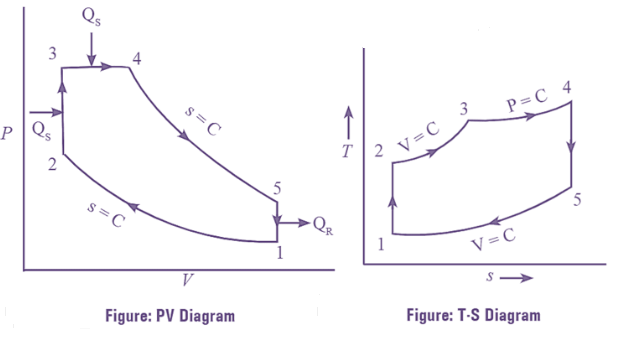The Dual cycle consists of four processes:

1. Isentropic Compression: The working fluid, usually air, is drawn into the compressor where it is compressed isentropically, meaning that the entropy of the fluid remains constant during the process. This process increases the pressure and temperature of the fluid, which is shown as a vertical line on a p-v diagram and a horizontal line on a T-s diagram.
2. Heat Addition: The compressed air is then heated by combustion of fuel in a combustor. This process increases the temperature and entropy of the fluid, which is shown as a curved line on a T-s diagram.
3. Isentropic Expansion: The heated fluid then expands through a turbine, where it performs work on the turbine and its entropy remains constant during the process. This process decreases the pressure and temperature of the fluid, which is shown as a vertical line on a p-v diagram and a horizontal line on a T-s diagram.
4. Heat Rejection: Finally, the cooled fluid is rejected to the environment, typically through a heat exchanger or a radiator, where it gives up the remaining heat that was not converted into useful work. This process decreases the temperature and entropy of the fluid, which is shown as a curved line on a T-s diagram.

In conclusion, the Dual cycle is a highly efficient thermodynamic cycle that is used to power gas turbines, and it is characterized by its isentropic compression and expansion processes and its heat addition and rejection processes. Understanding the working principle of the Dual cycle is important in the design and optimization of gas turbine systems.

# Recall the comparison between Otto, Diesel, and Dual Cycle

The Otto cycle, Diesel cycle, and Dual cycle are three commonly studied thermodynamic cycles in the field of internal combustion engines. Each cycle has its own unique characteristics and operating principles, and a comparison between the cycles can provide insight into their relative performance and efficiency.

1. Otto Cycle: The Otto cycle is a thermodynamic cycle that describes the operation of a spark-ignition internal combustion engine, such as a gasoline engine. It is characterized by a constant-volume heat addition process and an isentropic compression and expansion process. The Otto cycle has a high compression ratio, typically between 8:1 and 12:1, which allows for efficient combustion of the fuel and air mixture.
2. Diesel Cycle: The Diesel cycle is a thermodynamic cycle that describes the operation of a compression-ignition internal combustion engine, such as a diesel engine. It is characterized by a constant-volume heat rejection process and an isentropic compression and expansion process. The Diesel cycle has a lower compression ratio, typically between 14:1 and 20:1, which allows for the air to be compressed to a high enough pressure for spontaneous ignition of the fuel.
3. Dual Cycle: The Dual cycle, also known as the Brayton cycle, is a thermodynamic cycle that describes the operation of a continuous flow heat engine, such as a gas turbine. It is characterized by isentropic compression and expansion processes and heat addition and rejection processes. The Dual cycle has a relatively low compression ratio, typically between 2:1 and 6:1, and relies on high-temperature combustion of fuel to drive the turbine.

In conclusion, each of the three cycles has its own strengths and weaknesses, and the choice between cycles depends on the specific application and design requirements. The Otto cycle is well-suited for gasoline engines, the Diesel cycle is well-suited for diesel engines, and the Dual cycle is well-suited for gas turbines. Understanding the differences between the cycles is important for the design and optimization of internal combustion engines and gas turbine systems.

# Recall the working principle of Reversed Carnot cycle with the help of p-v and T-s diagrams

The Reversed Carnot cycle is a theoretical thermodynamic cycle that operates in reverse of the Carnot cycle. The Reversed Carnot cycle can be used to understand the maximum possible efficiency of a heat engine that operates between two temperature reservoirs.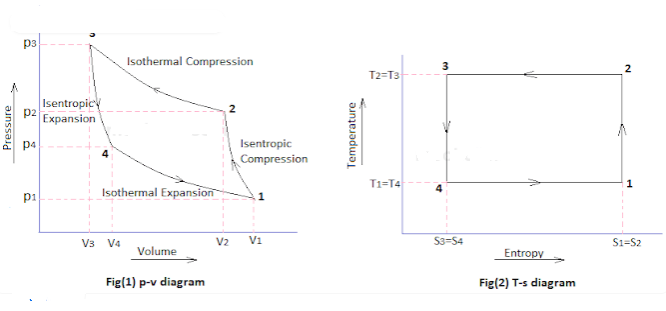The Reversed Carnot cycle consists of four processes:

1. Isothermal Expansion (I-E): The working fluid is expanded at a constant temperature T2. During this process, the pressure and volume of the fluid increase and work is done on the fluid.
2. Adiabatic Expansion (A-E): The working fluid is expanded adiabatically (without transfer of heat) to a lower temperature T1. The pressure and volume of the fluid continue to increase, but no work is done in this process.
3. Isothermal Compression (I-C): The working fluid is compressed at a constant temperature T1. During this process, the pressure and volume of the fluid decrease and work is done by the fluid.
4. Adiabatic Compression (A-C): The working fluid is compressed adiabatically to a higher temperature T2. The pressure and volume of the fluid decrease, but no work is done in this process.

The p-v and T-s diagrams of the Reversed Carnot cycle can be used to visualise the processes of the cycle and to understand the changes in the pressure, volume, temperature, and entropy of the working fluid. The p-v diagram represents the changes in pressure and volume of the working fluid, while the T-s diagram represents the changes in temperature and entropy of the working fluid. These diagrams are useful tools in understanding the thermodynamics of the Reversed Carnot cycle and in calculating its thermodynamic parameters, such as thermal efficiency and work done.

# Recall the working principle of Bell Coleman or Reversed Brayton cycle with the help of p-v and T-s diagrams

The Bell Coleman cycle, also known as the Reversed Brayton cycle, is a type of thermodynamic cycle used in refrigeration and air conditioning systems. The working principle of the Bell Coleman cycle can be represented on a p-v diagram and a T-s diagram.

On a p-v diagram, the cycle starts at a high pressure (point 1), where the refrigerant is in a high-pressure, high-temperature state. The refrigerant then enters an isentropic expansion process, where it undergoes a rapid decrease in pressure while its temperature decreases slightly (point 2 to 3). This process is often represented by an isentropic expansion line on a p-v diagram.

Next, the refrigerant enters a heat rejection process (point 3 to 4), where it gives off heat to the surrounding environment at a lower temperature. This process is often represented by a constant pressure line on a p-v diagram.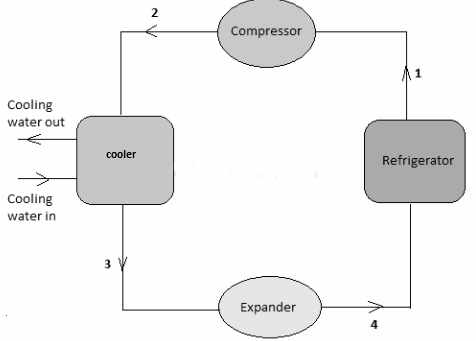After the heat rejection process, the refrigerant enters a isentropic compression process (point 4 to 1), where it undergoes a rapid increase in pressure while its temperature increases slightly. This process is often represented by an isentropic compression line on a p-v diagram.

On a T-s diagram, the Bell Coleman cycle can be represented by a series of lines connecting the various points of the cycle. These lines show the change in temperature and entropy of the refrigerant during each process of the cycle.

The Bell Coleman cycle is widely used in refrigeration and air conditioning systems due to its ability to efficiently transfer heat from a low-temperature environment to a high-temperature environment. This makes it a key component in many industrial, commercial, and residential heating, ventilation, and air conditioning (HVAC) systems.

# Derive an expression for COP of the Bell Coleman cycle

The coefficient of performance (COP) of the Bell Coleman cycle is a measure of the efficiency of the cycle, which is defined as the ratio of the useful work output to the heat input. It represents how much energy is converted into work compared to the energy input.

To derive an expression for COP of the Bell Coleman cycle, we need to consider the energy balance of the cycle. The heat input to the cycle is equal to the change in internal energy of the working fluid, which can be expressed as Q1-Q2, where Q1 is the heat input to the cycle and Q2 is the heat rejection from the cycle. The work output from the cycle is equal to the net work done by the working fluid, which can be expressed as Wnet. The COP of the Bell Coleman cycle can then be calculated as:

COP = Wnet / (Q1-Q2)

where Wnet is the network output of the cycle and Q1-Q2 is the heat input to the cycle. The COP of the cycle is a function of the temperatures of the heat sources and heat sinks, and the efficiency of the components in the cycle, such as the compressor and the turbine.

It is important to note that the higher the COP, the more efficient the cycle is in converting heat into work. The COP of the Bell Coleman cycle is often compared to that of the Carnot cycle, which is considered to be the most efficient cycle possible and sets a theoretical upper limit for the COP of any real cycle.

# Recall the working principle of the Rankine cycle with the help of p-v and T-s diagrams

The Rankine cycle is a thermodynamic cycle that is widely used in power plants to produce electricity from heat. It is a closed cycle, which means that the working fluid is cycled through the system without losing or gaining any substance, and the heat energy is transformed into mechanical energy through a turbine, which is then converted into electrical energy.

The Rankine cycle is based on the basic principles of thermodynamics and involves four processes, which are:

1. Pumping: The working fluid, typically water or steam, is pumped from a low-pressure region to a high-pressure region, increasing its pressure and temperature.
2. Heating: The high-pressure fluid is then heated to a higher temperature in a boiler, where heat is added to the fluid, causing its temperature to rise.
3. Expansion: The heated fluid then expands through a turbine, which converts the thermal energy into mechanical energy. During this process, the pressure and temperature of the fluid decrease.
4. Cooling: The low-pressure, low-temperature fluid is then cooled in a condenser, where it gives up heat to the surroundings and returns to the initial state of the cycle, completing the cycle.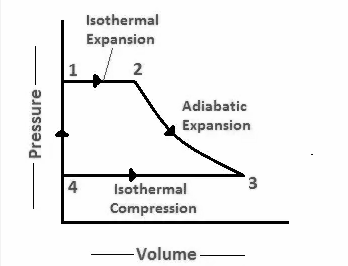A p-v diagram and a T-s diagram can be used to represent the Rankine cycle and to understand its working principle. In a p-v diagram, the vertical axis represents pressure, and the horizontal axis represents volume. The four processes of the cycle are represented by four lines on the p-v diagram. The T-s diagram, on the other hand, represents the cycle in terms of temperature and entropy. The vertical axis represents temperature, and the horizontal axis represents entropy.

The Rankine cycle is one of the most widely used thermodynamic cycles, due to its high efficiency, simplicity, and versatility. By optimising the parameters of the cycle, such as the pressure and temperature of the fluid, the efficiency of the Rankine cycle can be improved, making it a crucial component in the production of electricity in power plants.

# Derive the expression for the Efficiency of the Rankine cycle

The Rankine cycle is a thermodynamic cycle that is commonly used in steam power plants. It is a simple cycle that converts heat into work using steam as the working fluid. The efficiency of the Rankine cycle is a measure of how much of the heat input is converted into useful work.

The efficiency of the Rankine cycle can be expressed using the equation:

η = (Wnet / Qin) * 100%

Where:

η is the efficiency of the cycle, expressed as a percentage.

Wnet is the net work done during the cycle.

Qin is the total heat input into the cycle.

The net work done during the cycle is equal to the work done by the steam as it expands from the high-pressure state to the low-pressure state. The total heat input into the cycle is the heat added to the working fluid to increase its temperature from the low-temperature state to the high-temperature state.

To calculate the efficiency of the Rankine cycle, one must know the pressure, volume, and temperature at each state in the cycle. The temperature of the working fluid at the high-temperature state should be as high as possible, while the temperature of the working fluid at the low-temperature state should be as low as possible to maximise the efficiency of the cycle. The pressure ratio and the temperature ratio between the high and low-temperature states are key parameters that affect the efficiency of the Rankine cycle.